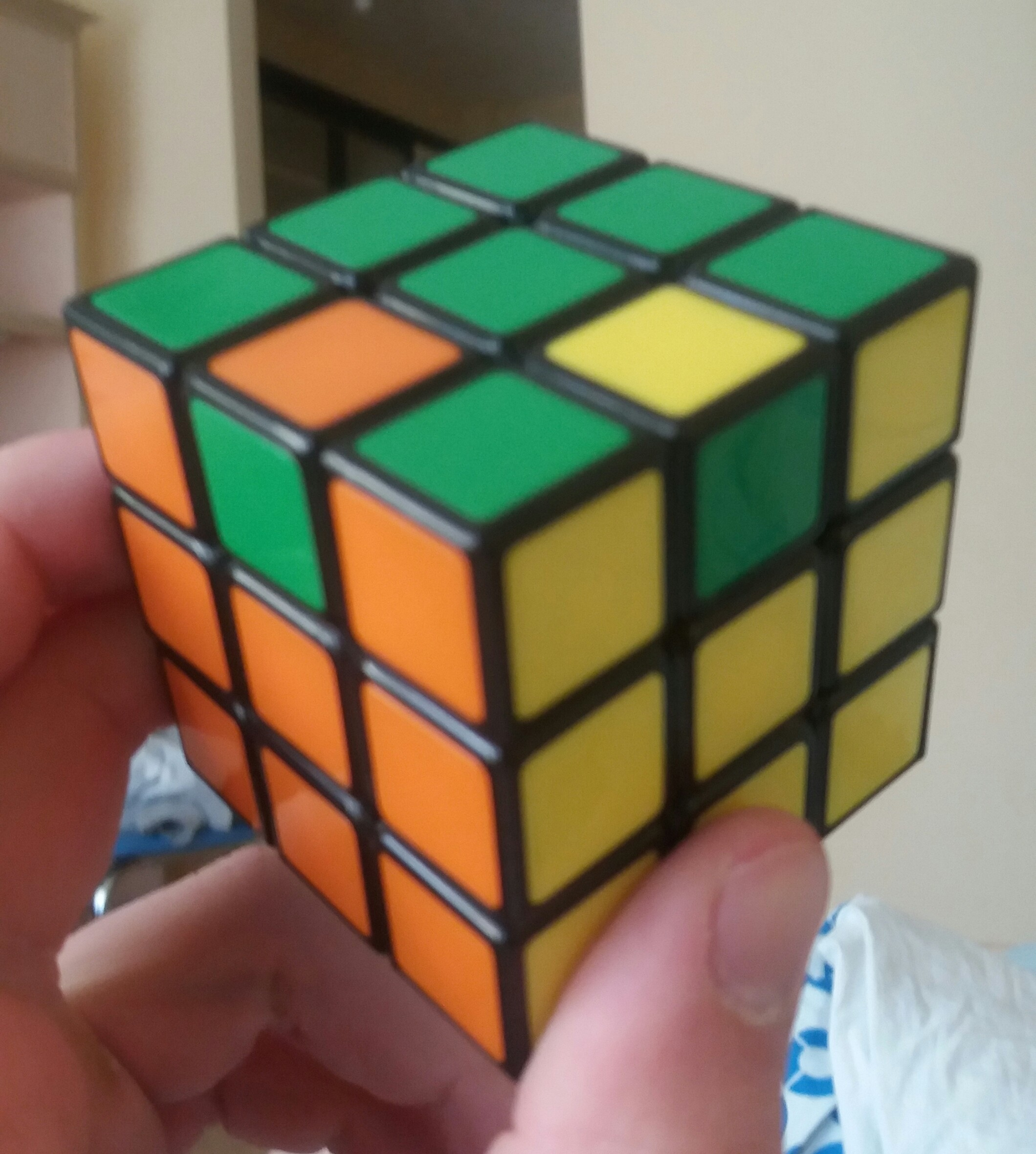# Can a Rubik's cube be put in a position not solvable by Fridrich's algorithm?

I think today I have faced a bug on rubik's cube. As it should be, I followed the steps Cross and then F2L, instead of OLL algorithms, I used R'FRF' and three corner swapping moves to complete last layer. After a long period, I saw that all corners and 2/4 edges solved correctly. There is not an algorithm to swap two edges. Is it possible ?

In 11 days we have passed I got an original cube. I have been trying to reach same position for 11 days so I did it today. This time I took photos and if it is needed I can upload more.• Your Rubik's cube is not in a solvable position. This state is equivalent to swapping two corners: it cannot be done, starting from a solved state. Is it possible someone's messing with you?
– user20
Mar 18, 2016 at 21:13
• Actually it is in a solvable position, I have figured out how I dit it. Following scramble on a solved cube gets you this position. F2 U M' U2 M U F2 M U' M' U2' M U' M'
– rekt
Mar 18, 2016 at 21:59
• That algorithm is a 3-cycle of two adjacent edges on the top layer, and one edge on the bottom layer, and can't swap two edges.
– user20
Mar 18, 2016 at 22:11
• Oh, sorry - I'm reading this position wrong. Yes, this position is solvable, but it's a different position than the one you originally had before your edit. The one in your original question isn't solvable, while the position in your edit is.
– user20
Mar 18, 2016 at 22:27
• Note that the image in the solver and the photo are not the same position. The solver screenshot has two edges swapped, which is not possible with valid moves. The photo has 2 edges flipped which is possible. Sep 22, 2020 at 16:17

There is no route from this position to a solved cube, using any algorithm. The reason is fairly simple: two edges cannot be swapped on a Rubik's cube. You can only swap edges in groups of three, called "cycles." (You may have heard the term 3-cycle before, and that's what it refers to.)

Along the same vein, this position can't be reached via any scramble. (If we could get there, we could get back.) As a result, you'll never see a position like this come up, if you start solved. Some positions are not possible on a Rubik's cube.

As a result, I suppose, technically there are positions which can't be solved by Fridrich's algorithm, but you can't reach them if you start from a solved cube.

Short answer is - No not by face turns alone from a solvable cube, but yes you may "put" the edges in a position that can not be solved by any algorithm that uses face turns alone (which would include Fridrich's algorithm).

If you were assembling a Rubik's cube you would have:

1. A frame of three axes with the six centre faces on the ends of each axis;
2. Eight corner pieces; and
3. Twelve edge pieces.

You could then choose where to put each piece in the frame and in which orientation to put each piece. The first corner may go in one of eight locations, the second in one of seven, and so on until there is only one possible location for the last corner piece and each can be placed in one of three orientations. Similarly the first edge may go in one of twelve locations, the second in one of eleven, and so on and each can be placed in one of two orientations.

There are, therefore, $8!*3^8*12!*2^{12}=519,024,039,293,878,272,000$ ways to put the cube together, however:

• $1/2$ of the ways you could place all the edges will be such that one edge is flipped relative to the others,
• $2/3$ of the ways you could place all the corners will be such that one corner is twisted relative to the others.
• $1/2$ of the ways you could place all the edges and corners together will have two corners swapped or, equivalently, two edges swapped (as in your case).

So only $1/2/3/2=1/12$ of the ways you could put the cube together will yield a solvable cube.
(Hence there are $8!*3^7*12!*2^{10}=43,252,003,274,489,856,000$ valid states)

The reason for your case is that the parity of the permutations generated by face turns is even.

One way to transform your posted cube from having two edges swapped to having two corners swapped would be to perform the following 11 turn transformation, given that U is white and F is blue:
R' U2 L' B' L U2 R' F R' F' R'